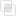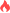PHP实现简单的加减乘除

php2021-07-31 16:27:12497浏览 · 0收藏 · 0评论private是私有的意思，该关键词表示私有的类成员，即只能被其定义所在的类访问。如上述例子中，我们声明了一个声明一个私有的方法。

public是公有的意思：公有的类成员可以在任何地方被访问。

```class MyCalculator {

private \$_fval, \$_sval;

public function __construct( \$fval, \$sval ) {

\$this->_fval = \$fval;

\$this->_sval = \$sval;

}

return \$this->_fval + \$this->_sval;

}

public function subtract() {

return \$this->_fval - \$this->_sval;

}

public function multiply() {

return \$this->_fval * \$this->_sval;

}

public function divide() {

return \$this->_fval / \$this->_sval;

}

}

\$mycalc = new MyCalculator(12, 6);

"; // 显示18

echo "相乘等于".\$mycalc-> multiply()."
"; // 显示72

echo "相减等于".\$mycalc-> subtract()."
"; // 显示6

echo "相除等于".\$mycalc-> divide()."
"; // 显示 2```php json中文不编码的实现方法php将word转换为html格式的方法php中nts是什么php设置SESSION_ID的方法最新PHP面试问题的总结PHP和Go进行环路链表检测php do-while循环语句的整理php函数引用传递参数的方法php函数按值传递参数的方法php检查某个日期是否有效php创建一个函数的方法php数组中改变指针位置的函数php引用类名的方法php命名空间的调用顺序php计算数组长度的方法css中skew函数的使用230

css中rotate函数是什么198

css中scale函数如何使用345